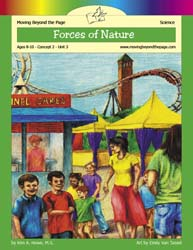# Forces of Nature Age 8-10: Concept 2 - Force and Power: Unit 3In this introduction to physics, explore force and motion. Perform experiments that demonstrate the laws of motion. Illustrate each of the different forms of energy. Understand the force of gravity. Explore the forces involved in natural events such as earthquakes and volcanoes. Create a slideshow or poster presentation to explain the various forces of nature.

This unit can be used independently but is designed to be taught in conjunction with the literature unit for The BFG by Roald Dahl.

## Prerequisites

• Able to write an organized paragraph
• Usually used by children in fourth grade

• Lesson 1: Physics and Force (2 Days)
• Lesson 2: Friction (2 Days)
• Lesson 3: Laws of Motion (2 Days)
• Lesson 4: Gravity
• Lesson 5: Projectiles
• Lesson 6: Energy (2 Days)
• Lesson 7: Pressure and Force Change the Environment (2 Days)
• Final Project: Poster or Presentation

## Summary of Skills

Moving Beyond the Page is based on state and national standards. These standards are covered in this unit.
• Connect science concepts, the history of science, and contributions of scientists. (Science)
• Determine factors that affect motion including force, friction, inertia, and momentum. (Science)
• Determine the motion of an object by following and measuring its position over time. (Science)
• Evaluate the impact of research on scientific thought, society, and the environment. (Science)
• Explain how energy is needed to make machines move. (Science)
• Identify and analyze forces that cause change in landforms. (Science)
• Measure and record changes in an object to which a force has been applied. (Science)
• Measure and record changes in an object to which a force such as a push or pull has been applied. (Science)
• Recognize factors that affect motion: force, friction, inertia, and momentum. (Science)
• Recognize forms of energy and how they are used. (Science)
• Recognize that forces such as earthquakes and glaciers can change the surface of the Earth. (Science)
• Recognize the different forms of energy and how they are used. (Science)
• Represent the natural world using models and illustrations. (Science)
• Represent the natural world using models. (Science)
• Create timelines. (Social Studies)
• Describe how individuals, events, and ideas have changed communities over time. (Social Studies)
← go back